# Information Theory

### ENTROPY / INFORMATION GAIN

Entropy - lack of order or lack of predictability (excellent slide lecture by Aurelien Geron)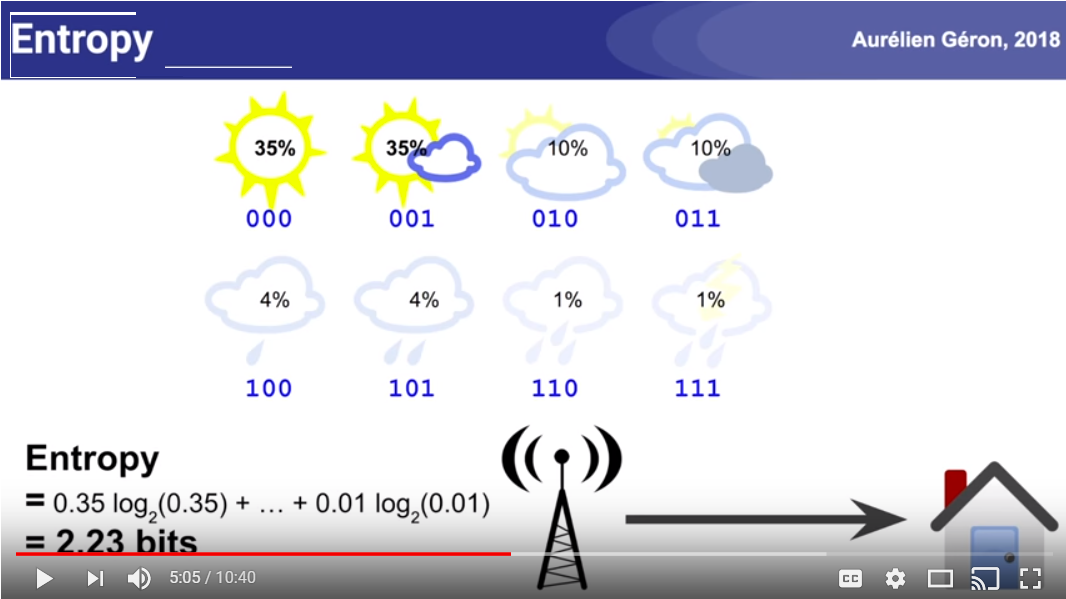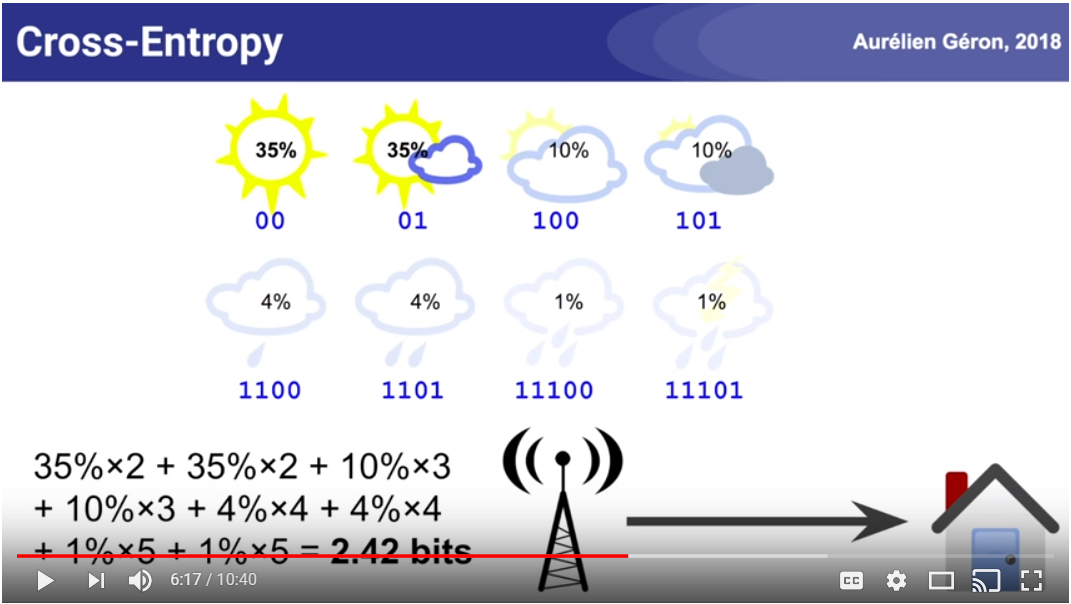Cross entropy will be equal to entropy if the probability distributions of p (true) and q(predicted) are the same. However, if cross entropy is bigger (known as relative_entropy or kullback leibler divergence)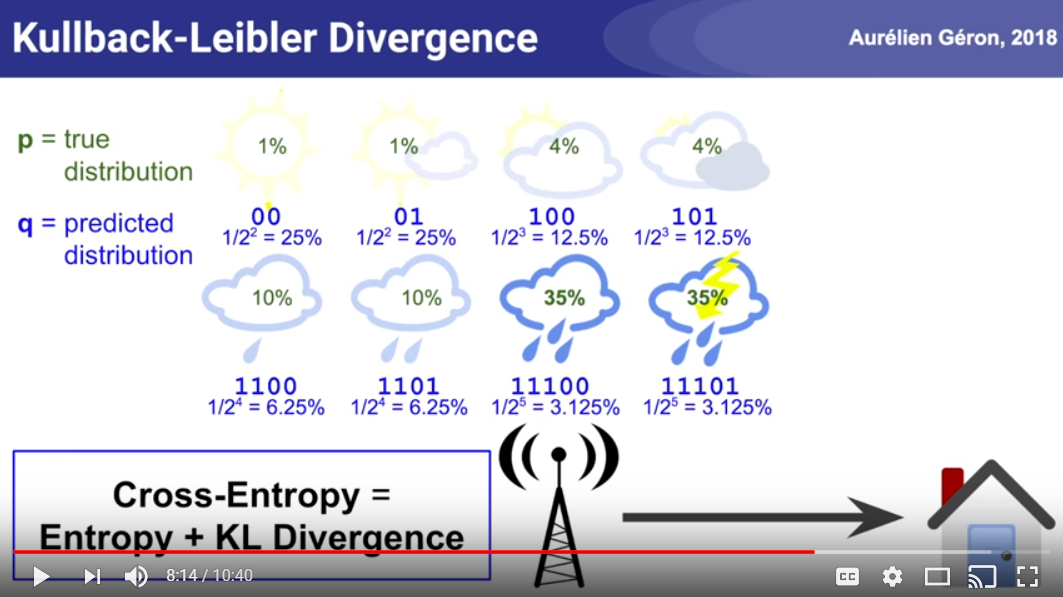In this example we want the cross entropy loss to be zero, i.e., when we have a one hot vector and a predicted vector which are identical, i.e., 100% in the same class for predicted and true, we get 0. In all other cases we get some number that gets larger if the predicted class probability is lower than zero as seen here: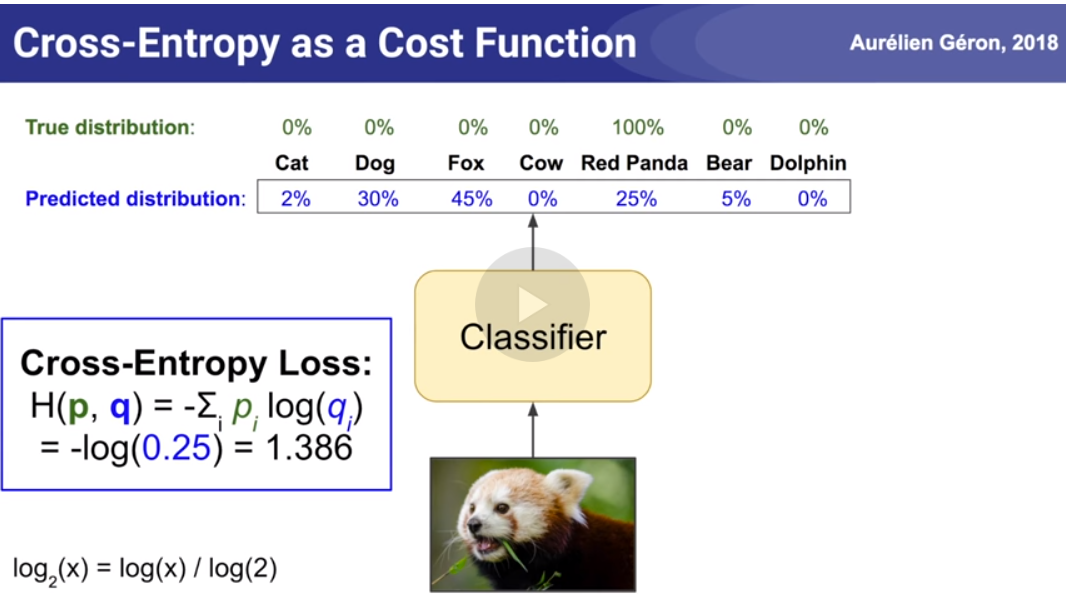Formula for 2 classes: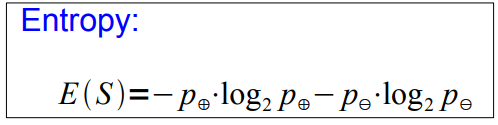NOTE: Entropy can be generalized as a formula for N > 2 classes: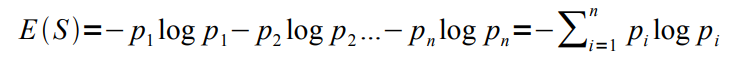(We want to grow a simple tree) awesome pdf tutorial→ a good attribute prefers attributes that split the data so that each successor node is as pure as possible
• i.e., the distribution of examples in each node is so that it mostly contains examples of a single class
• In other words: We want a measure that prefers attributes that have a high degree of „order“:
• Maximum order: All examples are of the same class
• Minimum order: All classes are equally likely → Entropy is a measure for (un-)orderedness Another interpretation:
• Entropy is the amount of information that is contained
• all examples of the same class → no information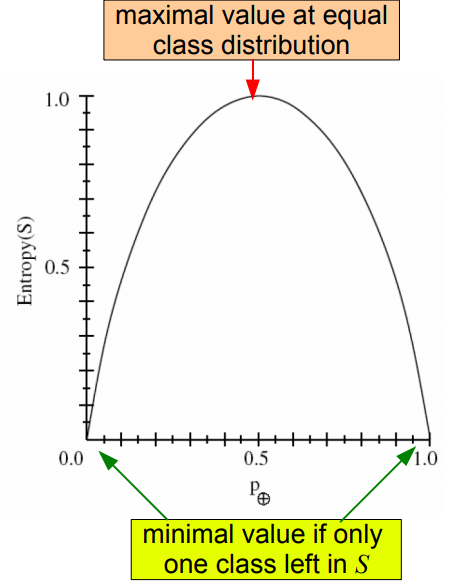Entropy is the amount of unorderedness in the class distribution of S
IMAGE above:
• Maximal value when the equal class distribution
• Minimal value when only one class is in S
So basically if we have the outlook attribute and it has 3 categories, we calculate the entropy for E(feature=category) for all 3.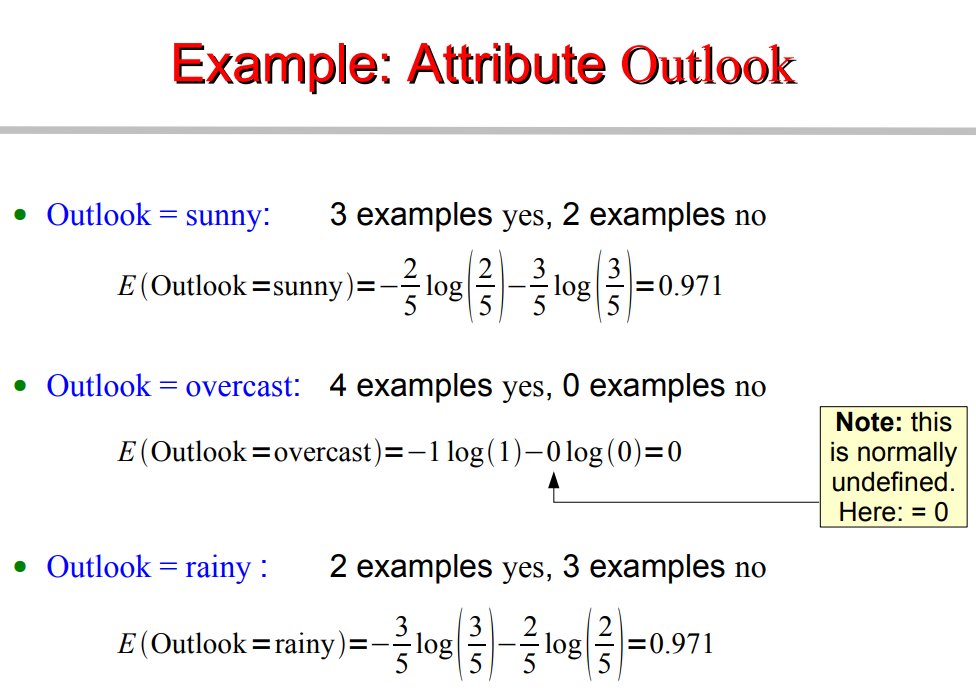INFORMATION: The I(S,A) formula below.
What we actually want is the average entropy of the entire split, that corresponds to an entire attribute, i.e., OUTLOOK (sunny & overcast & rainy)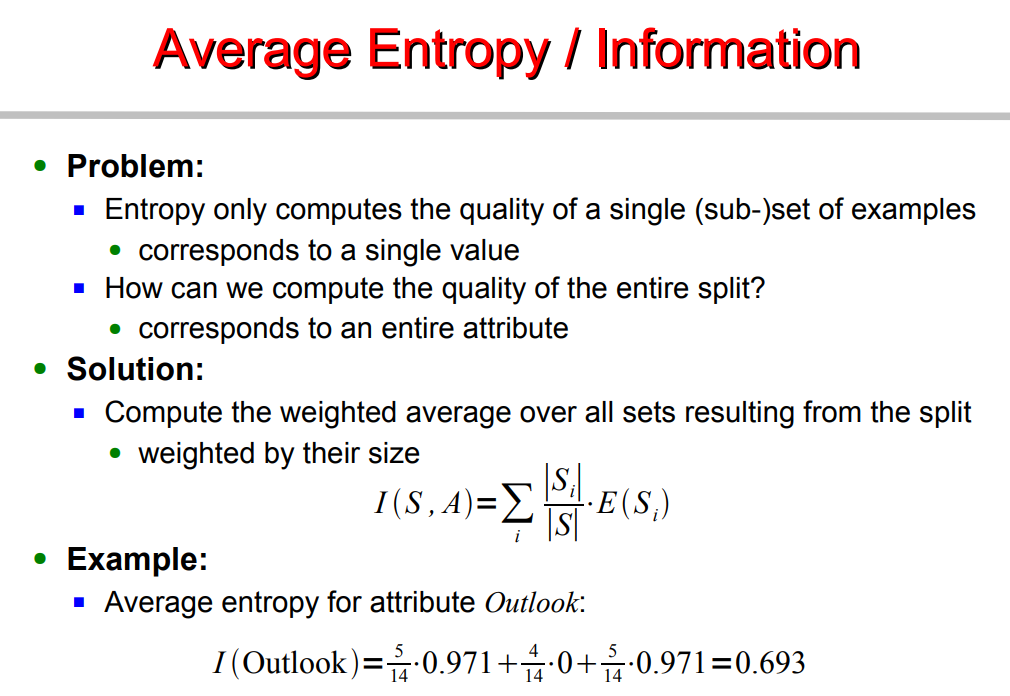Information Gain: is actually what we gain by subtracting information from the entropy.
In other words we find the attributes that maximizes that difference, in other other words, the attribute that reduces the unorderness / lack of order / lack of predictability.
The BIGGER GAIN is selected.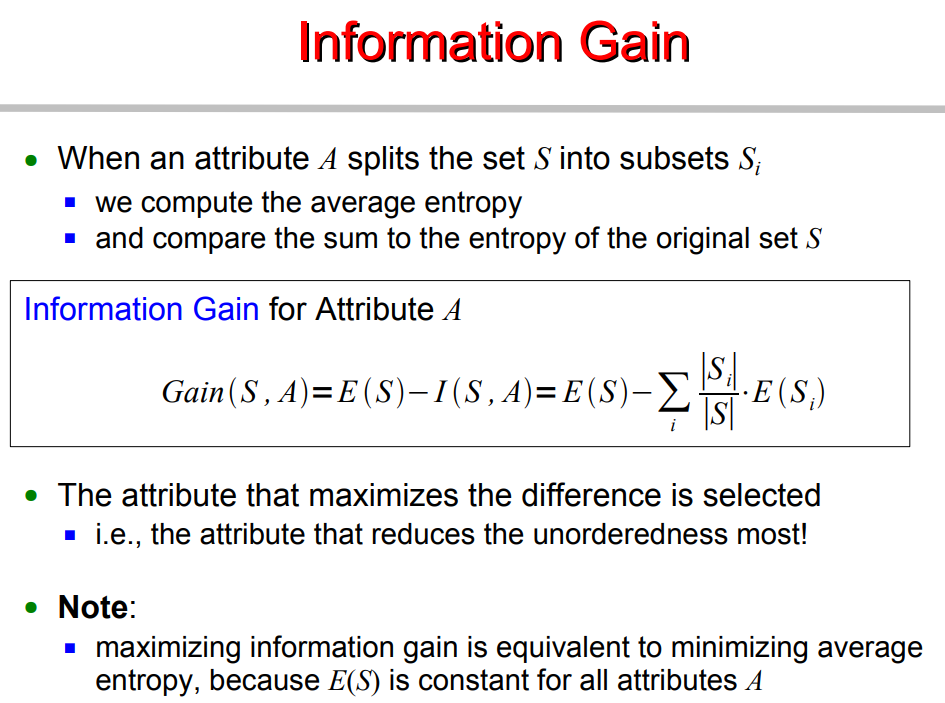There are some properties to Entropy that influence INFO GAIN (?):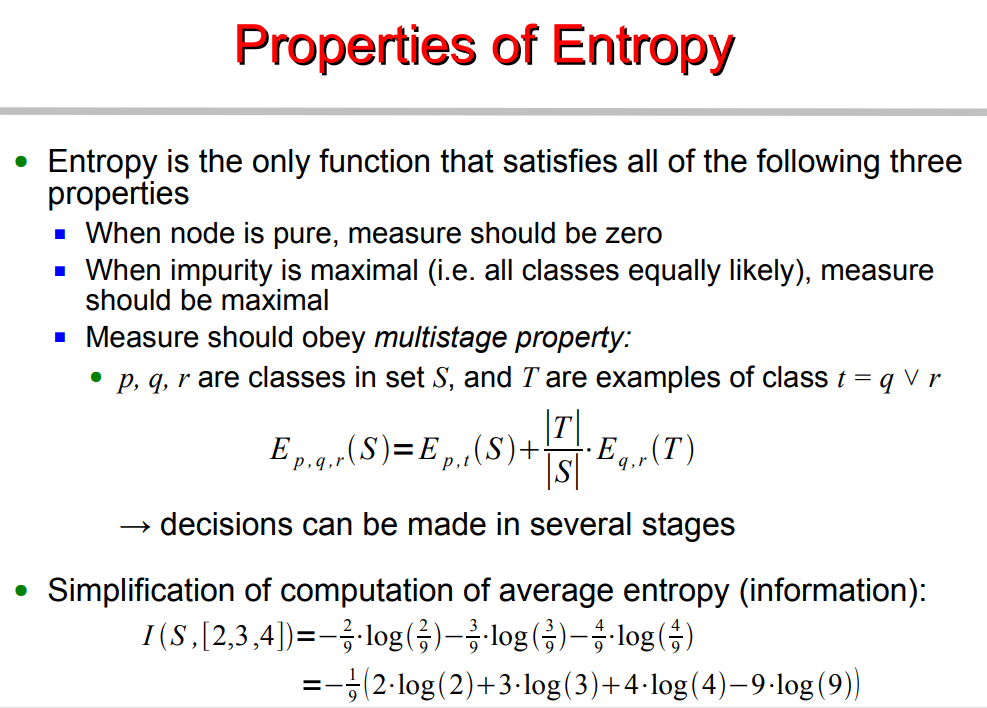There are some disadvantages with INFO GAIN, done use it when an attribute has many number values, such as “day” (date wise) 05/07, 06/07, 07/07..31/07 etc.
Information gain is biased towards choosing attributes with a large number of values and causes:
• Overfitting
• fragmentationWe measure Intrinsic information of an attribute, i.e., Attributes with higher intrinsic information are less useful.
We define Gain Ratio as info-gain with less bias toward multi value attributes, ie., “days”
NOTE: Day attribute would still win with the Gain Ratio, Nevertheless: Gain ratio is more reliable than Information Gain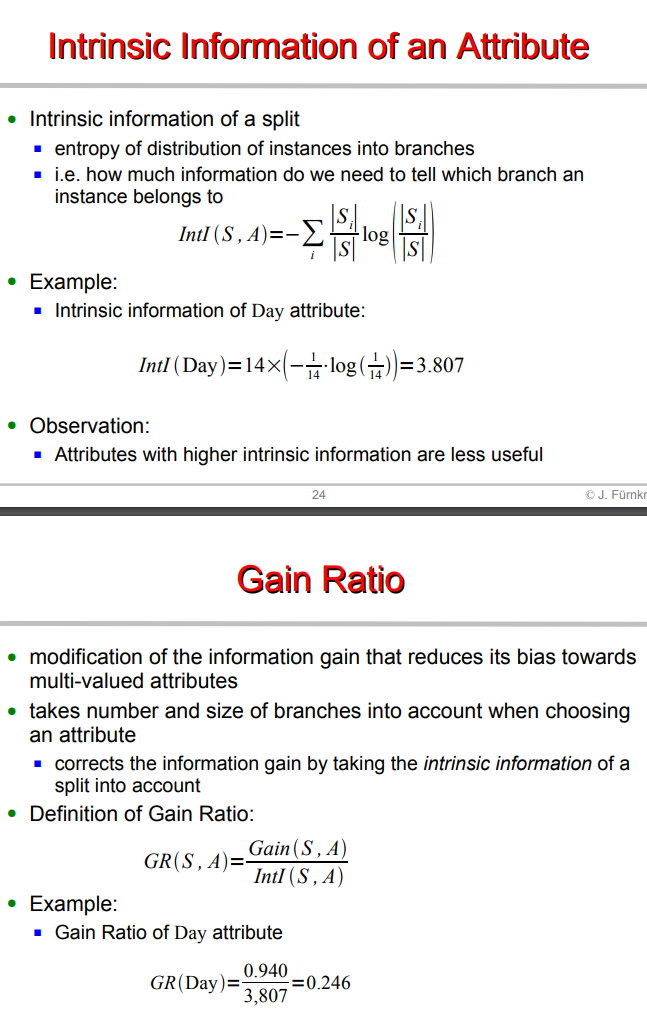Therefore, we define the alternative, which is the GINI INDEX. It measures impurity, we define the average Gini, and the Gini Gain.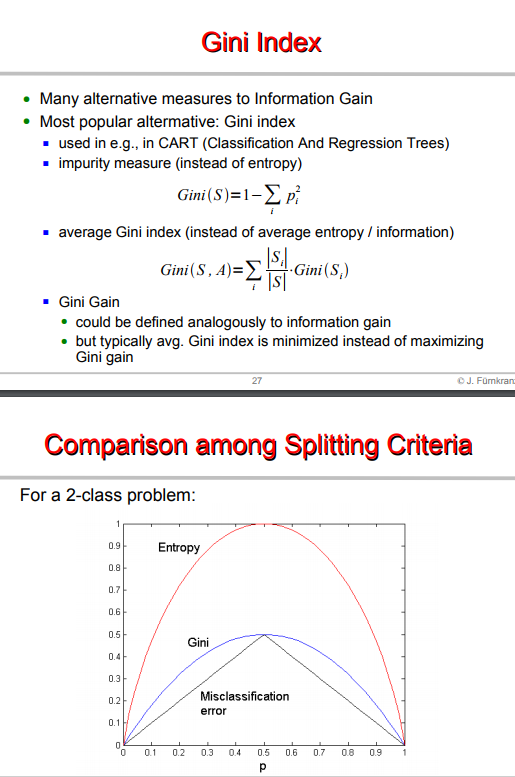### CROSS ENTROPY, RELATIVE ENT, KL-D, JS-D, SOFT MAX

1. 4.
Mastery on entropy, kullback leibler divergence (asymmetry), jensen-shannon divergence (symmetry) (has code)
2. 6.
Gensim on divergence metrics such as KL jaccard etc, pros and cons, lda is a mess on small data.
3. 7.

### SOFTMAX

1. 3.
Softmax vs cross entropy - Softmax loss and cross-entropy loss terms are used interchangeably in industry. Technically, there is no term as such Softmax loss. people use the term "softmax loss" when referring to "cross-entropy loss". The softmax classifier is a linear classifier that uses the cross-entropy loss function. In other words, the gradient of the above function tells a softmax classifier how exactly to update its weights using some optimization like gradient descent.
The softmax() part simply normalises your network predictions so that they can be interpreted as probabilities. Once your network is predicting a probability distribution over labels for each input, the log loss is equivalent to the cross entropy between the true label distribution and the network predictions. As the name suggests, softmax function is a “soft” version of max function. Instead of selecting one maximum value, it breaks the whole (1) with maximal element getting the largest portion of the distribution, but other smaller elements getting some of it as well.
This property of softmax function that it outputs a probability distribution makes it suitable for probabilistic interpretation in classification tasks.
Cross entropy indicates the distance between what the model believes the output distribution should be, and what the original distribution is. Cross entropy measure is a widely used alternative of squared error. It is used when node activations can be understood as representing the probability that each hypothesis might be true, i.e. when the output is a probability distribution. Thus it is used as a loss function in neural networks which have softmax activations in the output layer.

### TIME SERIES ENTROPY

1. 1.
entroPY - EntroPy is a Python 3 package providing several time-efficient algorithms for computing the complexity of one-dimensional time-series. It can be used for example to extract features from EEG signals.
print(perm_entropy(x, order=3, normalize=True)) # Permutation entropy
print(spectral_entropy(x, 100, method='welch', normalize=True)) # Spectral entropy
print(svd_entropy(x, order=3, delay=1, normalize=True)) # Singular value decomposition entropy
print(app_entropy(x, order=2, metric='chebyshev')) # Approximate entropy
print(sample_entropy(x, order=2, metric='chebyshev')) # Sample entropy
print(lziv_complexity('01111000011001', normalize=True)) # Lempel-Ziv complexity
1. 1.
PyInform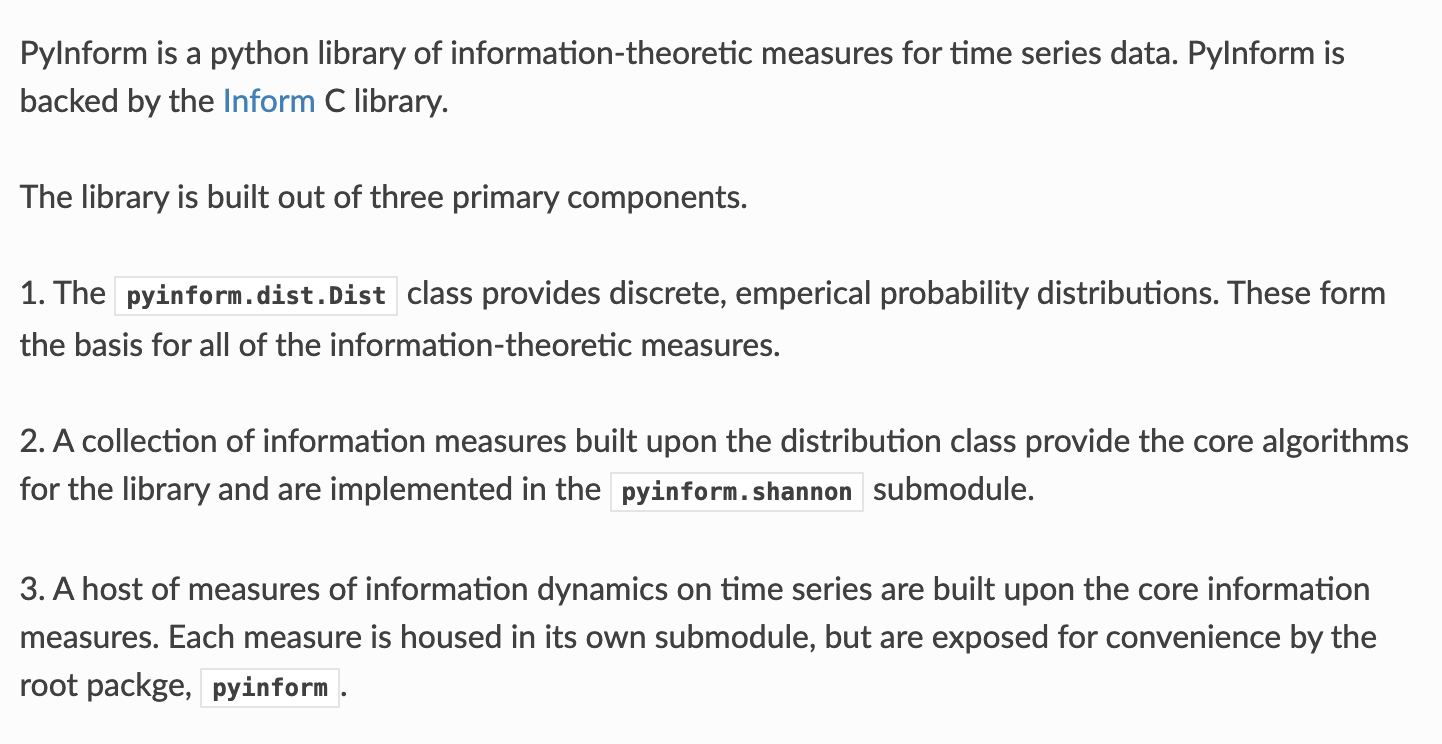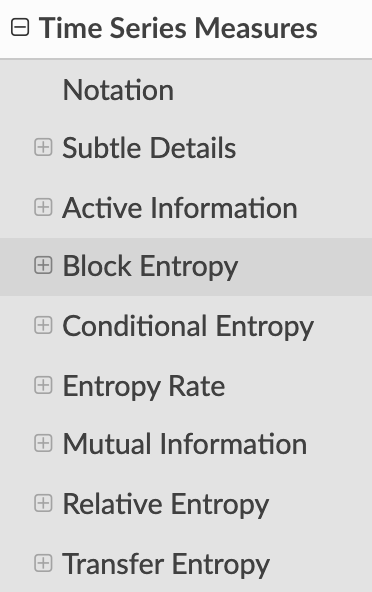### Complement Objective Training

1. 1.
Article by LightTag, paper -
COT is a technique to effectively provide explicit negative feedback to our model. The technique gives us non-zero gradients with respect to incorrect classes, which are used to update the model's parameters.
COT doesn't replace cross-entropy. It's used as a second training step as follows: We run cross-entropy, and then we do a COT step. We minimize the cross-entropy between our target distribution. That's equivalent to maximizing the likelihood of the correct class. During the COT step, we maximize the entropy of the complement distribution. We pretend that the correct class isn't an option and make the remaining classes equally likely.
But, since the true class is an option, and we're training for it explicitly, maximizing the true classes probability and pushing the remaining classes to be equally likely is actually pushing their probabilities to 0 explicitly, which provides explicit gradients to propagate through our model.